Next: 2.3.3.5 Linear Inductor Up: 2.3.3 Devices Previous: 2.3.3.3 Linear Capacitor

### 2.3.3.4 Non-linear Capacitor

The constitutive relation for a non-linear capacitor is I = dQ/dt. Using the backward Euler discretization scheme to discretize dQ/dt one obtains
 I =(2.37)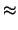G . V -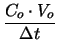(2.38) G =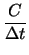(2.39)

Equation (2.38) is a commonly used approximation which, however, does not guarantee charge conservation [66,67]. For constant C (2.38) of course simplifies to (2.35). The stamp is given as
 yx, y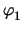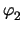f n1 G - G - I n2 - G G I

Tibor Grasser
1999-05-31